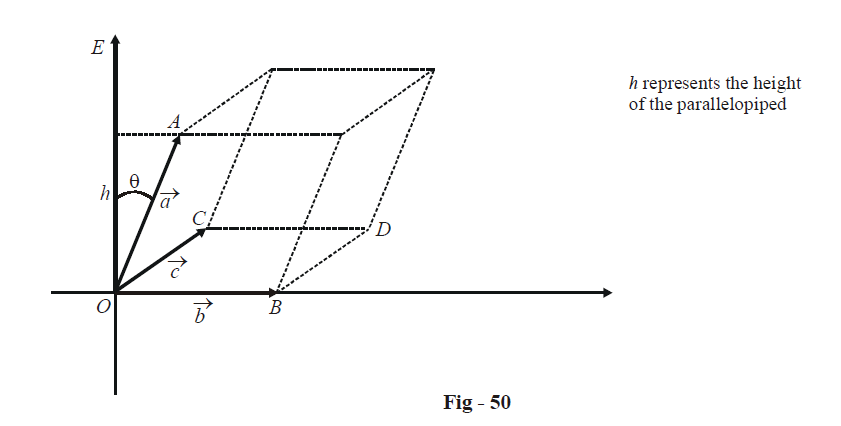# Scalar Triple Product Of Vectors

Go back to  'Vectors and 3-D Geometry'

As the name suggests, a scalar triple product involves the (scalar) product of three vectors. How may such a product be defined?

Consider three vectors $$\vec a,\,\,\vec b\,\,{\text{and}}\,\vec c$$ . Consider the quantity  $$\vec a \cdot (\vec b \cdot \vec c)$$ . Since  $$\vec b \cdot \vec c$$  is a scalar, you cannot define its dot product with another vector. Thus,$$\vec a \cdot (\vec b \cdot \vec c)$$ is a meaningless quantity.

However, consider the expression $$\vec a \cdot (\vec b \times \vec c)$$. Since $$\vec b \times \vec c$$ is a vector, its dot product with $$\vec a$$ is defined. Thus, $$\vec a \cdot (\vec b \times \vec c)$$ is defined and is termed the scalar triple product of $$\vec a,\,\,\vec b\,and\,\vec c.$$ This product is represented concisely as $$[\vec a\;\;\vec b\;\;\vec c]$$.

An alert reader might have noticed that another valid triple product is possible:$$\vec a \times (\vec b \times \vec c)$$ . This is the vector triple product and is considered in the next section.

Let us try to assign a geometrical interpretation to the scalar triple product (STP)  $$[\vec a\;\;\vec b\;\;\vec c]$$.

First of all, make $$\vec a,\;\;\vec b,\;\;\vec c$$ co-initial. Assume for the moment that  $$\vec a,\;\;\vec b,\;\;\vec c$$ are non-coplanar. Complete the parallelopiped with $$\vec a,\;\;\vec b,\;\;\vec c$$ as adjacent edges:Consider $$\vec b \times \vec c$$. This is a vector perpendicular to the plane containing $$\vec b\,\,{\text{and}}\,\,\vec c$$  . We have represented it by $$\overrightarrow {OE}$$ . Let the angle between $$\vec a$$ and $$\overrightarrow {OE}\;$$be $$\theta$$. What can $$\vec a \cdot (\vec b \times \vec c)$$ i.e.$$\vec a \cdot \overrightarrow {OE}$$ represent ? $$\left| {\overrightarrow {OE} } \right|$$  represents the area of the parallelogram OBDC.

Thus,

\begin{align}&\vec a \cdot \overrightarrow {OE} = \left| {\vec a} \right|\;\left| {\overrightarrow {OE} } \right|\cos {\theta } \hfill \\\\&\qquad\;\;= (\left| {\vec a} \right|\cos {\theta })\;\overrightarrow {OE} \hfill \\\\&\qquad\;\; = {\text{ }}\left( {Height{\text{ }}of{\text{ }}the{\text{ }}parallelopiped\,h} \right){\text{ }} \times {\text{ }}\left( {Area{\text{ }}of{\text{ }}the{\text{ }}base{\text{ }}parallelogram} \right) \hfill \\\\&\qquad\;\; = {\text{ }}Volume{\text{ }}of{\text{ }}the{\text{ }}parallelopiped. \hfill \\ \end{align}

\boxed{\begin{align}&{\text{The}}\;\,STP\;\,[\vec a\;\vec b\;\vec c]\;\,{\text{therefore}}\;\,{\text{represents}}\;\,{\text{the}}\;\,{\text{volume}}\;\, \hfill \\&{\text{of}}\;{\text{the}}\;\,{\text{parallelopiped}}\;{\text{with}}\;\vec a,\;\vec b,\;\vec c\;{\text{as}}\;{\text{adjacent}}\;{\text{edges}} \hfill \\ \end{align}}

Note that the volume V of the parallelopiped could equally well have been specified as

$V = \vec b \cdot (\vec c \times \vec a) = [\vec b\;\vec c\;\vec a]$

$= \vec c \cdot (\vec a \times \vec b) = [\vec c\;\vec a\;\vec b]$

Thus, we come to an important property of the STP:

$[\vec a\;\vec b\;\vec c] = [\vec b\;\vec c\;\vec a] = [\vec c\;\vec a\;\vec b]$

that is, if the vectors are cyclically permuted, the value of the STP remains the same. However, note that

\begin{align}&[\vec a\;\;\vec b\;\vec c]= \vec a \cdot (\vec b \times \vec c) \hfill \\\\& \qquad\quad= - \vec a \cdot (\vec c \times \vec b) \hfill \\\\&\qquad\quad = - [\vec a\;\;\vec c\;\;\vec b] \hfill \\ \end{align}

Vectors
grade 11 | Questions Set 1
Vectors